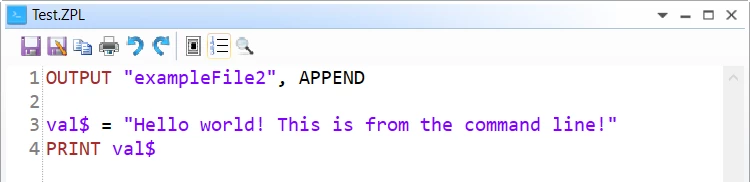Solved

# Exporting to .txt file when running a macro from the command line

• 5 replies
• 218 views• Monochrome
• 4 replies

I am currently tyring to run a macro from the command line of my computer. The macro includes the command :

>>> OUTPUT 'exampleFile', APPEND

This is used to create an output txt file by the name of 'exampleFile.' Now, when I run this macro through the command line, no file is generated. But, when this macro is run through optic studio, there is a file that is generated named exampleFile. I don't understand why this step is ignored when the macro is used through the command line?

icon

Best answer by Allie 17 August 2020, 21:53

View original

### 5 replies

Userlevel 6+2

Hi Isaiah,

I have tested this in OpticStudio 20.2.2, and I do not see the same behavior. When I run my macro through the command line, a file is created. If the file already exists, then the contents are edited with the output of my macro. Here is the macro I wrote:

OUTPUT 'exampleFile2', APPEND

val\$ = 'Hello world! This is from the command line!'

PRINT val\$

Here are the commands I used in the command line:

cd C:\Program Files\Zemax OpticStudio 20.2.2

OpticStudio.exe -zpl='C:\Users\Alexandra.Culler\Desktop\Zemax\Macros\Test.ZPL'

Are you using a similar process? Can you let me know what you are writing in the command line? Also, have you been able to get any other macro commands to work through the command line?

Thanks,

AllieHi Allie,

Thanks for your reply. These are the exact steps I have been using as well unforutnately. I have also added the 'BEEP' command to confirm that the macro has finished running. So, I am able to get other commands to work, but unfortunately not the OUTPUT command. I am running OpticStudio_20.1 though... could this possibly be an issue?Hmm... Now I am confused because I was actually able to get your code to save the file from the command line so it must not be a version issue. Here is the code I am using. Do I have the output syntax correct? (The ouput line is in bold to find easier)

! Define initial variables

surfTotalNum = NSUR() # find total number of surfaces

mirror\$ = 'MIRROR' # Set mirror string variable

code_coda = OCOD('CODA') # Obtain the code from the Coda Operand

!###################################################################################

!Loop through system and perform calculations

! Also tried puting the output command here

! OUTPUT 'ExampleFile', APPEND

FOR i, 0, surfTotalNum, 1

glassType\$ = \$GLASS(i)

IF (glassType\$ \$== mirror\$)

##############################################################################

!Define Variables

surf_num = i

wave_num = 1

field_num = 1

px = 0

py = .5

##############################################################################

#calculates S polarization

v1 = OPEV(code_coda, surf_num, wave_num, field_num, -6, px, py)

v2 = OPEV(code_coda, surf_num, wave_num, field_num, -7, px, py)

prod1 = v1 * v1

prod2 = v2 * v2

summ_s = prod1 + prod2

##############################################################################

#calculates P polarization

v1 = OPEV(code_coda, surf_num, wave_num, field_num, 6, px, py)

v2 = OPEV(code_coda, surf_num, wave_num, field_num, 7, px, py)

prod1 = v1 * v1

prod2 = v2 * v2

summ_p = prod1 + prod2

##############################################################################

summ_tol = summ_p + summ_s

reflection_tol = summ_tol / 2

##############################################################################

# Use this section for exporting to txt file in proper format

OUTPUT 'ExampleFile', APPEND

print i

print summ_s

print summ_p

print reflection_tol

ENDIF

NEXT

BEEP

Userlevel 6+2

Hi Isaiah,

I did test in 20.1 and can confirm that the method should work there.

Actually, it looks like my code was re-formatted a bit. Whenever we declare a string, it should be surrounded in quotation marks. When I pasted the code here, the quotation marks were switched to apostrophes. Sorry about that! Here is the code as I see it in OpticStudio:Actually, I just tried to run your code. I think I found the problem! When we run a macro through the command line in the way I presented above, OpticStudio is opened with a blank lens file and then the macro is run. As your code is now, the OUTPUT keyword is within an IF statment which is searching for a MIRROR surface. A blank lens file will not have a MIRROR surface, so everything within the IF statement will be ignored.

When we use the OUTPUT keyword on line 13 (with the correct formatting), then the file will be created as expected, but it will be blank.

As a test, add a LOADLENS command to the top of your macro so that the lens file of interest is opened with the macro! Let me know how that works!

Best,

AllieOh goodness that was it! Completely forgot to load the lens haha. Thank you so much for your help!!